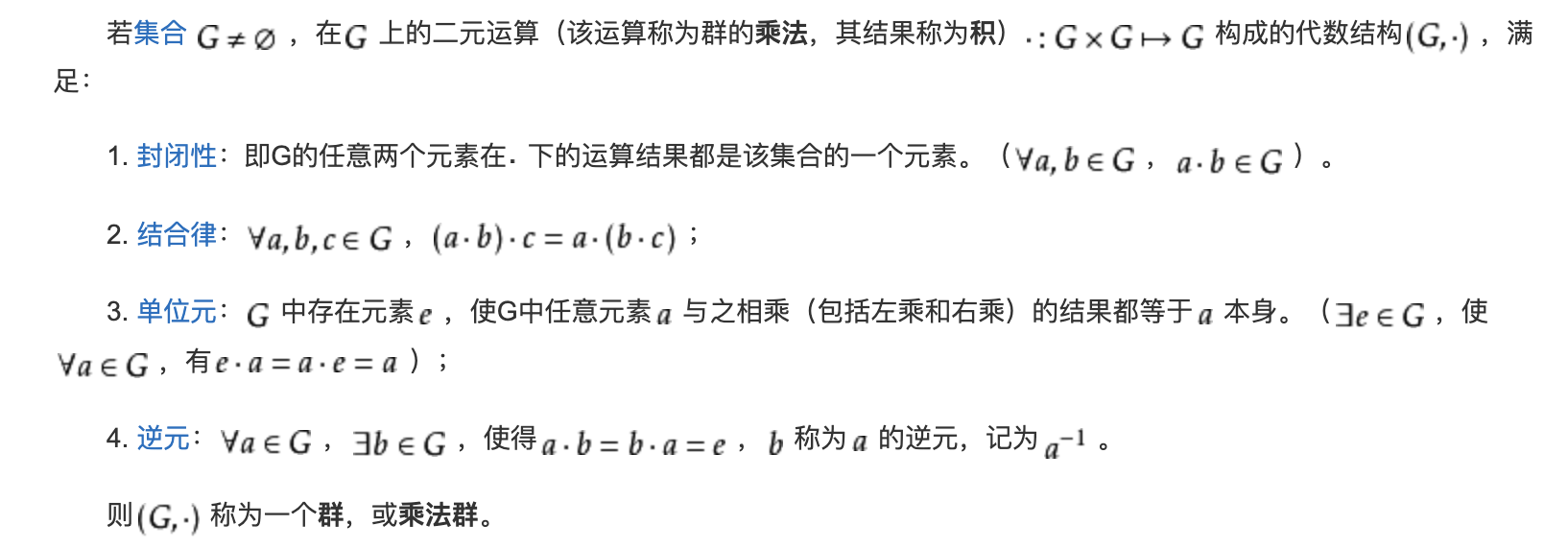# Shamir 秘密共享方案和Feldman可验证的秘密共享方案

### 常用的秘密共享方案

安全多方计算的研究主要是针对无可信第三方的情况下，如何保证各方数据安全的同时利用多方数据进行计算，得到预期结果。
安全多方计算不是指某一单独的协议, 而是一些关键技术集合，比如：秘密共享、混淆电路、不经意传输等等。

今天来记录一下学习Shamir 秘密共享方案 和Feldman可验证的秘密共享方案的笔记。

#### 首先提出问题——平均工资问题

A、B、C、D 如果在不暴露自己工资的情况下，求得四人的平均工资？

最简单的方案如下：
1，A生成一个随机数，将其与自己的工资相加，用B的公钥加密发送给B
2，B用自己的私钥解密，加进自己的工资，然后用C的公钥加密发送给C
3，C用自己的私钥解密，加进自己的工资，然后用D的公钥加密发送给D
4，D用自己的私钥解密，加进自己的工资，然后用A的公钥加密发送给A
5，A用自己的私钥解密，减去原来的随机数得到工资总和
6，A将工资总和除以人数得到平均工资，宣布结果

存在问题：

该协议假定所有参与者都是诚实的不会谎报工资，A不谎报结果。

如何保证节点诚实？且A不谎报结果？
一般的解决方案是运用比特承诺协议让A向B传送他的随机数但这种方案会导致协议结束后，B知道了A的工资。

其他还有什么更好的方案吗？
秘密分享！

#### 什么是秘密共享？

秘密共享是信息安全和数据保密中的重要手段，它在重要信息和秘密数据的安全保存、传输及合法利用中起着非常关键的作用。
秘密共享由两个算法——秘密份额的分配算法和秘密的恢复算法构成。
在执行秘密份额的分配算法时，分发者将秘密分割成若干份额在一组参与者中进行分配，使得每个参与者都得到关于该秘密的一个秘密份额；
秘密的恢复算法保证只有参与者的一些特定的子集才能有效的恢复秘密，其他子集不能有效地恢复秘密，甚至得不到关于秘密任何有用信息。

最早被提出的秘密共享模型是门限类的秘密共享模型，Shamir 秘密共享方案就是一种门限秘密共享模型。
$\ (t,n)$ 门限秘密共享体制中，秘密的持有者，把秘密分割成$\ n$ 个份额，分别分配于$\ n$ 个参与者，使得只要获得这$\ n$ 个份额中的至少$\ t$个才可能有效地恢复出原来的秘密，而任何少于$\ t$ 个的份额集合都不能恢复出原来的秘密，得不到关于原秘密任何有用的信息。

#### Shamir秘密共享分案 算法流程

一共$\ n$个节点（$\ p_1$,$\ p_2$,…,$\ p_n$）拥有自己的秘密值（$\ v_i$ ），如何计算这$\ n$个节点秘密的和，即求$\ v_1$+$\ v_2$+$\ v_3$+…+$\ v_n$

基本思路： n个节点，各自将秘密分成n分，发给参与计算的其余n-1个节点，随后，节点收到来自其他节点的秘密碎片，加上自己的共n个，计算碎片的和，将所得的和再次发给其他参与计算的节点，等待收到t个来自其他节点的和之后即可得出最后结论。

步骤一：随机值的共享 ，每个节点拥有均需一份相同的$\ X$={$\ x_1$,$\ x_2$,…,$\ x_n$}
步骤二：各节点$\ p_i$分别随机选择一个$\ t-1$ 次的多项式$\ f_i$ ( $\ f_i(x)$ = $\ a_i$ $\ x^{t-1}$ + $\ b_i$$\ x^{t-2}$ + $\ c_i$$\ x^{t-3}$ +…+$\ v_i$ ），因此这里加上$\ v_i$ 在内一共产生了$\ t$ 个未知数（$\ a_i， b_i$,…），这 $\ t$ 个数是只有当前节点才会知道的，且每个节点都会随机产生不同的$\ t$ 个未知数，其中$\ t-1$ 个随机数，1个秘密值。
步骤三：各节点$\ p_i$根据自己的多项式$\ f_i$ 和随机值$\ X$确定一组共享值$\ f_i(x_1)$ ,$\ f_i(x_2)$ ,$\ f_i(x_3)$ ,…,$\ f_i(x_n)$ ,分别发给节点$\ p_1$$\ p_2$，…，$\ p_n$
步骤四：各节点$\ p_i$将自己收到的结果（$\ f_1(x_i)$$\ f_2(x_i)$$\ f_3(x_i)$、…、$\ f_n(x_i)$）相加得到 $\ R_i$=($\ a_1$ +$\ a_2$ + $\ a_3$+… +$\ a_{n}$)$\ x^{t-1}_i$+ ($\ b_1$ +$\ b_2$ + $\ b_3$+… +$\ b_{n}$)$\ x^{t-2}_i$ + … + ( $\ v_1$ +$\ v_2$ + $\ v_3$+… +$\ v_{n}$) ，并将结果发送给其他节点。
步骤五：节点收到的$\ R_i(i=0,1,...,t)$ 放在一起就是一个$\ t$ 元 [ ($\ a_1$ +$\ a_2$ + $\ a_3$+… +$\ a_{n}$)、($\ b_1$ +$\ b_2$ + $\ b_3$+… +$\ b_{n}$) 、…、 ( $\ v_1$ +$\ v_2$ + $\ v_3$+… +$\ v_{n}$) ]的方程组，节点收到多于等于$\ t$ 个节点的$\ R$结果就能解出 ( $\ v_1$ +$\ v_2$ + $\ v_3$+… +$\ v_{n}$)。

通过分析算法流程可得：
这种方式攻击者只有同时获取了步骤一中的共享的随机值$\ X$ 及大于等于$\ t$ 个步骤四中的$\ R$ 才能得到原密码值。

不足：
Shamir秘密共享其实是包含了一些可能不切实际的假设的——秘密的分发者和参与者都是诚实的，然而, 在现实生活中, 参与者可能是不诚实, 他可能会故意或是由于一些非主观因素（如网络传输错误 ）提供了错误的份额, 这样会导致也无法正确地恢复秘密。

#### 可验证秘密共享的提出

为了检验共享的正确性,出现了可验证的秘密共享方案。 一个正常执行的可验证秘密共享方案能够保证:在秘密分发阶段, 分发者发送给参与者的共享是正确的;在秘密恢复阶段, 参与者提交的共享也是正确的。

Feldman可验证的秘密共享方案和pederson可验证秘密共享方案是最早出现的也是现在应用最多的两个秘密共享方案，其中Feldman可验证秘密共享方案是最早提出的不需要可信机构的计算安全的秘密共享方案，而pederson是最早提出的无条件安全的秘密共享方案。

本文只介绍Feldman。

#### 什么是计算安全？什么是无条件安全？

评估密码系统安全性主要有三种方法：
（1）无条件安全性
这种评价方法考虑的是假定攻击者拥有无限的计算资源，但仍然无法破译该密码系统。
（2）计算安全性
这种方法是指使用目前最好的方法攻破它所需要的计算远远超出攻击者的计算资源水平，则可以定义这个密码体制是安全的。
（3）可证明安全性
这种方法是将密码系统的安全性归结为某个经过深入研究的数学难题（如大整数素因子分解、计算离散对数等），数学难题被证明求解困难。这种评估方法存在的问题是它只说明了这个密码方法的安全性与某个困难问题相关，没有完全证明问题本身的安全性，并给出它们的等价性证明。

介绍Feldman可验证的秘密共享方案前，先介绍一些 涉及到的密码学的基本符号和概念：
a｜b：表示a能整除b
群：（截图来自百度百科）mod运算：模运算，a mod n是a除以n的余数，模运算支持一些算术运算，本文会用到的是： (a mod p * b mod p) mod p = (a * b) mod p

#### Feldman可验证的秘密共享

算法参数设置 : p、q是大素数、且有q | (p-1）、g是 乘法群 $\Z^{*}_q$ 中的一个 p阶元素，这些参数都是公开的。
具体协议如下（在Shamir秘密共享分案的基础上）:

1. 份额分发阶段:每个节点$\ p_i$分发份额之后，计算并广播承诺 {$\alpha_i = g^{param_i}$ mod q } （$\ g$ 的次数$\ param_i$为步骤二中所选的多项式的参数, $\ a_{i}$,$\ b_{i}$,$\ c_{i}$ ，…，一共$\ t$个值）。

2. 共享验证:每个节点 $\ p_i$检验它所接受的$f_j(x_i) (j=0,1,...,n)$是否满足等式：$\ g^{f_j(x_i)} =\prod^{t-1}_{j=0}\alpha_j^{x_i}$ mod q 。如果等式不成立, 那么节点 $\ p_i$ 所收到的共享$\ f_j(x_i)$是无效的。
等式为什么会成立：
$\prod^{t-1}_{j=0}\alpha_j^{x_i} mod q =(g^{param_0x^{t-1}_i} g^{param_1x^{t-2}_i} g^{param_3x^{t-3}_i} \cdots g^{v_j}) mod q = g^{(a_jx^{t-1}_i+b_jx^{t-2}_i+c_jx^{t-3}_i+v_j)} mod q = g^{f_j(x_i)} mod q$

3. 秘密恢复:每一参与者 $\ p_i$向其他参与者广播自己的共享$\ R_i$，当接受到的$\ R$ 值大于$\ t$ 个，且均被验证为有效时
$\prod^{t-1}_{j=0}\alpha_j^{x_i} \beta_j^{x_i} \gamma_j^{x_i} \cdots mod q =g^{R_i}$,$\alpha,\beta,\gamma,...$分别为节点$\ p$ 在份额分发阶段广播的承诺）, 计算出秘密。

这一方案可抵抗 (n-1) /2个恶意的参与者, 由于 $\alpha_{i}$被公开, 所以该方案只是计算安全的(根据密码学中的计算离散对数的困难性可得 $\alpha_{i} = g^{a_i}$ mod q 中已知g、$\alpha_{i}$ 和 q 是很难算出来 $\ a_i$ 的。

博主之前没接触过密码学，这只是我的学习笔记，有不对的地方烦请指出，不胜感激！

参考资料：
张福泰, 赵福祥, 王育民. 可验证秘密分享及其应用[J]. 电子学报, 2002, 30(10):1519-1525.
石润华, 黄刘生. 一种简单的可验证秘密共享方案[J]. 计算机应用, 2006(08):77-79.
https://blog.csdn.net/wangtingyao1990/article/details/79475842

还能输入1000个字符没有更多推荐了，返回首页

©️2019 CSDN 皮肤主题: 游动-白 设计师: 上身试试扫一扫，手机浏览